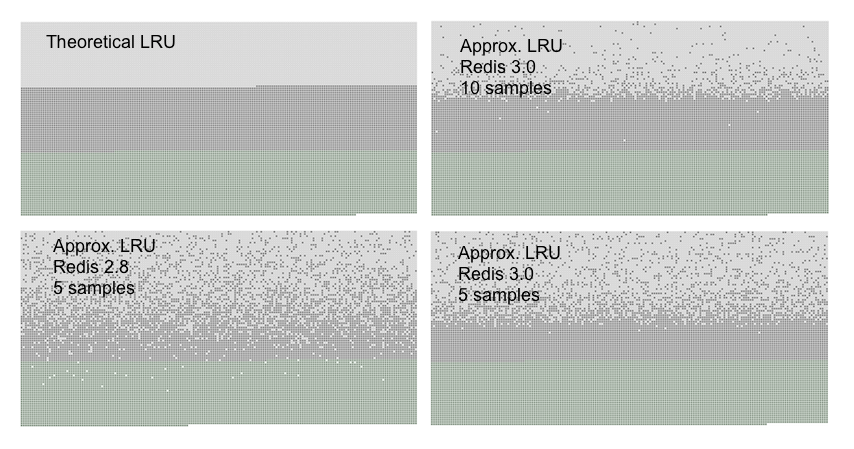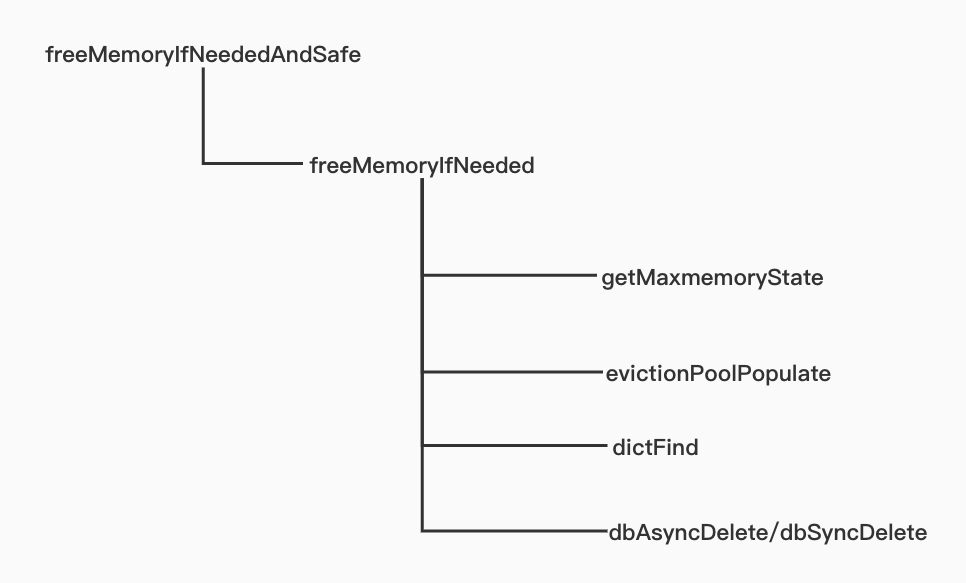# 走近源码：Redis如何清除过期key

2019/04/10 10:10

“叮……”，美好的周六就这么被一阵钉钉消息吵醒了。<!-- more -->

Redis中会保存一个“过期key池”，这个池子中存放了一些可能会被清理的key。其中保存的数据结构如下：

struct evictionPoolEntry {
unsigned long long idle;    /* Object idle time (inverse frequency for LFU) */
sds key;                    /* Key name. */
sds cached;                 /* Cached SDS object for key name. */
int dbid;                   /* Key DB number. */
};


### 近似LRU

LRU算法的缺点是，我们需要维护一个全部（或只有过期时间）key的列表，还要按照最近使用时间排序。这会消耗大量内存，并且每次使用key时更新排序也会占用额外的CPU资源。对于Redis这样对性能要求很高的系统来说是不被允许的。### 源码分析int getMaxmemoryState(size_t *total, size_t *logical, size_t *tofree, float *level) {
size_t mem_reported, mem_used, mem_tofree;

mem_reported = zmalloc_used_memory();
if (total) *total = mem_reported;

int return_ok_asap = !server.maxmemory || mem_reported <= server.maxmemory;
if (return_ok_asap && !level) return C_OK;

mem_used = mem_reported;

if (level) {
if (!server.maxmemory) {
*level = 0;
} else {
*level = (float)mem_used / (float)server.maxmemory;
}
}

if (return_ok_asap) return C_OK;

if (mem_used <= server.maxmemory) return C_OK;

mem_tofree = mem_used - server.maxmemory;

if (logical) *logical = mem_used;
if (tofree) *tofree = mem_tofree;

return C_ERR;
}


• total：已使用的字节总数，无论是C_OK还是C_ERR都有效。
• logical：已使用的内存减去slave或AOF缓冲区后的大小，只有返回C_ERR时有效。
• tofree：需要释放的内存大小，只有返回C_ERR时有效。
• level：已使用内存的比例，通常是0到1之间，当超出内存限制时，就大于1。无论是C_OK还是C_ERR都有效。

for (i = 0; i < server.dbnum; i++) {
db = server.db+i;
dict = (server.maxmemory_policy & MAXMEMORY_FLAG_ALLKEYS) ?
db->dict : db->expires;
if ((keys = dictSize(dict)) != 0) {
evictionPoolPopulate(i, dict, db->dict, pool);
total_keys += keys;
}
}


void evictionPoolPopulate(int dbid, dict *sampledict, dict *keydict, struct evictionPoolEntry *pool) {
int j, k, count;
dictEntry *samples[server.maxmemory_samples];

count = dictGetSomeKeys(sampledict,samples,server.maxmemory_samples);
for (j = 0; j < count; j++) {
unsigned long long idle;
sds key;
robj *o;
dictEntry *de;

de = samples[j];
key = dictGetKey(de);
/* some code */
if (server.maxmemory_policy & MAXMEMORY_FLAG_LRU) {
idle = estimateObjectIdleTime(o);
}

/* some code */
k = 0;
while (k < EVPOOL_SIZE &&
pool[k].key &&
pool[k].idle < idle) k++;
if (k == 0 && pool[EVPOOL_SIZE-1].key != NULL) {
continue;
} else if (k < EVPOOL_SIZE && pool[k].key == NULL) {
} else {
if (pool[EVPOOL_SIZE-1].key == NULL) {
sds cached = pool[EVPOOL_SIZE-1].cached;
memmove(pool+k+1,pool+k,
sizeof(pool)*(EVPOOL_SIZE-k-1));
pool[k].cached = cached;
} else {
k--;
sds cached = pool.cached; /* Save SDS before overwriting. */
if (pool.key != pool.cached) sdsfree(pool.key);
memmove(pool,pool+1,sizeof(pool)*k);
pool[k].cached = cached;
}
}
/* some code */
}
}


/* Go backward from best to worst element to evict. */
for (k = EVPOOL_SIZE-1; k >= 0; k--) {
if (pool[k].key == NULL) continue;
bestdbid = pool[k].dbid;

if (server.maxmemory_policy & MAXMEMORY_FLAG_ALLKEYS) {
de = dictFind(server.db[pool[k].dbid].dict,
pool[k].key);
} else {
de = dictFind(server.db[pool[k].dbid].expires,
pool[k].key);
}
/* some code */
if (de) {
bestkey = dictGetKey(de);
break;
}
}


if (server.lazyfree_lazy_eviction)
dbAsyncDelete(db,keyobj);
else
dbSyncDelete(db,keyobj)


delta -= (long long) zmalloc_used_memory();
mem_freed += delta;


### 清理策略

• noeviction：不会继续处理写请求（DEL可以继续处理）。
• allkeys-lru：对所有key的近似LRU
• volatile-lru：使用近似LRU算法淘汰设置了过期时间的key
• allkeys-random：从所有key中随机淘汰一些key
• volatile-random：对所有设置了过期时间的key随机淘汰
• volatile-ttl：淘汰有效期最短的一部分key

Redis4.0开始支持了LFU策略，和LRU类似，它分为两种：

• volatile-lfu：使用LFU算法淘汰设置了过期时间的key
• allkeys-lfu：从全部key中进行淘汰，使用LFU

0
0 收藏

### 作者的其它热门文章0 评论
0 收藏
0